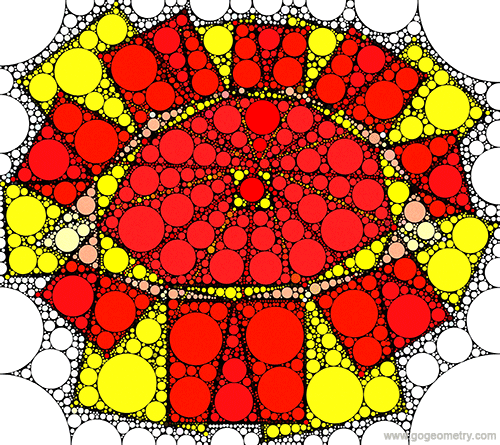# Geometry Problem 1325

### Elements: Octagons, Midpoints, Squares

In the figure, the yellow octagon B1B2...B8 is the midpoint octagon of an octagon A1A2...A8. The red octagon C1C2...C8 is the midpoint octagon of the yellow octagon B1B2...B8. O1, O2, ..., O8 are the centers of yellow squares on side B1B2, ... B8B1. Q1, Q2, ..., Q8 are the centers of red squares on sides C1C2, ..., C8C1. O15, O 26, O 37, O 48, Q15, Q26, Q37, and Q48 are midpoints of O1O5, O2O6, O3O7, O 4O8, Q1Q5, Q2Q6, Q3Q7, and Q4Q8, respectively. Prove that (1) Quadrilateral O15O26O37O48 and Q15Q26Q37Q48 are squares (2) Q15, Q26, Q37, and Q48 are the midpoints of O15O26, O26O37, O37O48, and O48O15, respectively.

The midpoint polygon of a polygon P is the polygon whose vertices are the midpoints of the sides of P.### Art of Geometry Problem 1325 using Mobile Apps, iPad. Circle Patterns

Geometric art is a form of art based on the use and application of geometric figures. A geometric figure is any set or combination of points, lines, surfaces and solids. A mobile app or mobile application software is a computer program designed to run on smartphones and tablet computers.